4k+1形式的奇数有：1,5,9,13,17,21,25,···。我们来看一下这些奇指标的斐波那契数。

u1=1，              1=4×0+1

u3=2=1×2，          1=4×0+1

u5=5=1×5，          5=4×1+1

u7=13=1×13，        13=4×3+1

u9=34=1×2×17，     17=4×4+1

u11=89=1×89，      89=4×22+1

u13=233=1×233，    233=4×58+1

u15=610=1×2×5×61，61=4×15+1

············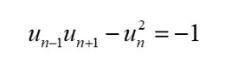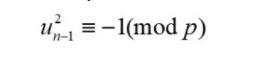一个正整数n被另一个正整数p除，余数可以是0(整除),1,2，···，p-1。若余数为p-1，我们也可以说余数为-1。记做：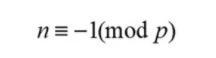比如，28除以13，带余除式为：28=2×23+2；若是25除以13，则带余除式可以写成：25=1×13+12，但也可以写成2×23-1。所以，25≡12(mod13)或25≡-1 (mod 13)。下面这个结论很重要：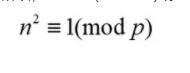更进一步，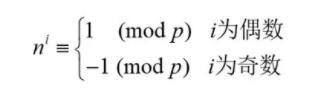这个结论的证明不复杂：把n≡-1 (mod p)写成下面第一式，从而有下面的其他无穷多的恒等式。看上面每一行的最后一项即常数项，即可得出结论，这是因为其他项都可以被p整除。这些常数项是间隔着的1和-1。

 费马小定理：若p是素数，n为不能被p整除的正整数，则能够被p整除。或者说，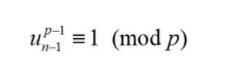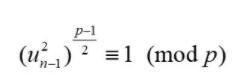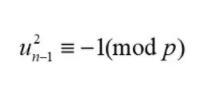p=4k+1